# Repeated series

(diff) ← Older revision | Latest revision (diff) | Newer revision → (diff)

A series whose terms are also series:(1)

The series (1) is said to be convergent if for any fixedthe seriesconverges and if also the series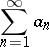converges. The sum of the latter is also called the sum of the repeated series (1). The sum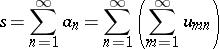of the repeated series (1) is the repeated limit of the partial sums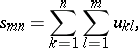i.e.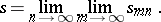If the double series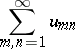converges and the series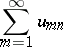converges, then the repeated series (1) converges and it has the same sum as the double series . The condition of this theorem is fulfilled, in particular, if the double series

converges absolutely.